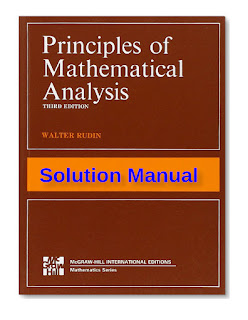APPLIED MATHEMATICS

# Solution Manual to Principles of Mathematical Analysis 3rd Edition PDF Free DownloadThis is a complete solution guide to all exercises in Rudin’s Principles of Mathematical Analysis. The features of this book are as follows: It covers all the 285 exercises with detailed and completed solutions. As a matter of fact, my solutions show every detail, every step and every theorem that I applied. There are 55 illustrations and 3 tables for explaining the mathematical concepts or ideas used behind the questions or theorems. Sections in each chapter are added so as to increase the readability of the exercises. Different colors are used frequently in order to highlight or explain problems, lemmas, remarks, main points/formulas involved, or show the steps of manipulation in some complicated proofs. (ebook only) Necessary lemmas with proofs and references are provided because some questions require additional mathematical concepts which are not covered by Rudin.